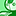# QlikView App Dev

Discussion Board for collaboration related to QlikView App Development.

Announcements
Become an analytics expert with Qlik's new 15 week course: Applied Data Analytics using Qlik Sense. READ MORE
cancel
Showing results for
Did you mean:Partner

## help with nested loop

Hi all,

I have the following loop within my script..

LET Dir = 'C:\Test1\Temp';

ODBC CONNECT32 TO [DBF Driver];

SET errormode = 0;

For each file in FileList('\$(Dir)\*.DBF')

LET vFilename = subfield(subfield('\$(file)', '\', -1),'.',1);

If len(subfield('\$(file)','\',-1))>12 then

LET Data = chr(96) & '\$(Dir)' & chr(96) & '\' & chr(96) & Subfield(GetShortName('\$(file)'),'\',-1) & chr(96);

else

LET Data = chr(96) & '\$(Dir)' & chr(96) & '\' & chr(96) & Subfield('\$(file)','\',-1) & chr(96);

ENDIF;

Data:

SQL SELECT *

FROM \$(Data);

SET errormode = 1;

STORE Data into ;

DROP TABLE Data;

NEXT file;

say i have multiple directories, what i want to do is loop through the multiple directories and perform the above loop in each.

e.g.

if my directories are :

C:\Test1\Temp

C:\Test2\Temp

C:\Temp\Test

how would i list those directories then get a loop to go to the first one, run the above loop then go to the 2nd one, run the above loop then again with the 3rd directory.

Any help would be appreciated.

Thanks

1 Solution

Accepted SolutionsSpecialist II

Wrap another loop around and change the Dir assignment like this ...

For each d in 'C:\Test1\Temp','C:\Test2\Temp','C:\Temp\Test'

Let Dir = '\$(d)';

{rest of current code}

next d;

flipsideSpecialist II

Wrap another loop around and change the Dir assignment like this ...

For each d in 'C:\Test1\Temp','C:\Test2\Temp','C:\Temp\Test'

Let Dir = '\$(d)';

{rest of current code}

next d;

flipsideCommunity Browser